Related Articles
Food Ordering System in C
• Last Updated : 20 Oct, 2020

Prerequisite: Modular Approach in Programming

Problem Statement: Write C program to implement Food ordering with the following functionalities:

• Login & Signup
• Search by Food
• Search by hotels
• Cart & Order Confirmation

Approach and Functionality:

### Signup Functionality

This is the main function for signup, From here we are calling the account_check() function to validate the inputs. Following are the functionalities:

• Input the username, age, email, password, confirm the password, and mobile number.
• Validate the inputs
• Username must contain alphabets.
• Age must be greater than and not equal to 0.
• Email must contain @, a comma and length should be greater than 5.
• Passwords must contain length between 8 to 12 with at least one uppercase, lowercase, number and special character. Both password and confirm password should be the same.
• Mobile number should contain numbers and exactly 10 digits.

After the successful validation of all input fields, it checks whether the same account already exists or not through account_check() functionality.

### Login Functionality

This function implements the login features of our project. While login, the Email, and Password are validated and checked whether it is already signed up. After the successful login, there is an option to choose either Search_by_food() or Search_by_hotels() functionalities.

### Order by Hotel

In this functionality, the food order is placed after selecting any Hotel. Once the hotel is selected the list of food is displayed with their respective costs. Once the food is selected, you need to go to option Select Cart for the successful ordering of the foods.

Before ordering by the hotel, initialize some default hotels which we are given locally through structures in C for our demonstration. Once the hotels and their respective foods are initialized dynamically, it will display the list of hotels.

### Order by Food

Once the hotel is selected in the above step, select the food and enter the number of items to order. Once the selection of all the foods is done it will display the total amount of food selected. Move to Select Cart for the successful ordering of the foods.

### Cart

This functionality perform the following:

• Display the total cost of your orders.
• Confirm your order and enjoy your food.

## Initialization

 `// C program to initialize the Food``// Ordering System``// Initialization``#include `` ` `// Structure to store the user details``// i.e., Signup details``struct` `details {``    ``char` `uname;``    ``int` `age;``    ``char` `password;``    ``char` `email;``    ``char` `mobile;``};`` ` `// Structure to store the hotels``// and their food details``struct` `hotels {``    ``char` `hotel;``    ``char` `first_food;``    ``char` `second_food;``    ``char` `third_food;``    ``char` `fourth_food;``    ``int` `first, second, third, fourth;``};`` ` `// Initialize the hotels and details``struct` `hotels m;``struct` `details s;`` ` `// Function to get the input for``// a new account``void` `signup();`` ` `// Function to check whether account``// is already existed or not``void` `account_check();`` ` `// Function to validate all input fields``int` `validate();``void` `login();``void` `cart();``void` `search_by_hotels();``void` `search_by_food();``void` `food_order(``int` `food);`` ` `// Function to initialize the hotels and``// food structure dynamically``void` `hotel_initialize();``void` `hotels(``int` `hotel_choice);`` ` `int` `flag = 1, i, j = 0, count = 0, caps = 0;``int` `small = 0, special = 0, numbers = 0;``int` `success = 0, x, choice;``char` `temp_name, temp_password1;``char` `temp_password2, temp_email;``char` `temp_mobile;`` ` `int` `temp_age, total = 0, food_choice, n;``int` `hotel_choice, search_choice, confirm;``int` `ch, food, hotel_id;`

## Boilerplate

 `// C program to implement Boilerplate``// Code for the Food Ordering System``int` `main()``{``    ``while` `(1) {``        ``printf``(``"\n\nWelcome to Food "``);`` ` `        ``printf``(``"Ordering System"``               ``"\n\n1)SIGNUP\n"``);``        ``printf``(``"2)LOGIN\n3)EXIT\n\n"``);``        ``printf``(``"Enter your choice\t"``);``        ``scanf``(``"%d"``, &choice);`` ` `        ``// Switch Cases``        ``switch` `(choice) {`` ` `        ``// For Signup Function``        ``case` `1: {``            ``signup();``            ``break``;``        ``}`` ` `        ``// For Login Function``        ``case` `2: {``            ``login();``            ``break``;``        ``}``        ``case` `3: {``            ``exit``(1);``        ``}``        ``default``: {``                ``printf``(``"\nPlease Enter the "``                ``printf``(``"valid choice\n"``);``                ``break``;``        ``}``        ``}``    ``}``}`

## Signup

 `// Function to create a new user for``// the Food ordering system``void` `signup()``{``    ``printf``(``"Enter Your name\t"``);``    ``scanf``(``"%s"``, temp_name);`` ` `    ``printf``(``"Enter Your Age\t"``);``    ``scanf``(``"%d"``, &temp_age);`` ` `    ``printf``(``"Enter Your Email\t"``);``    ``scanf``(``"%s"``, temp_email);`` ` `    ``printf``(``"Enter Password\t"``);``    ``scanf``(``"%s"``, temp_password1);`` ` `    ``printf``(``"Confirm Password\t"``);``    ``scanf``(``"%s"``, temp_password2);`` ` `    ``printf``(``"Enter Your Mobile Number\t"``);``    ``scanf``(``"%s"``, temp_mobile);`` ` `    ``// Function Call to validate``    ``// the user creation``    ``x = validate();``    ``if` `(x == 1)``        ``account_check();``}`

## Validate

 `// Function to validate the user for``// the signup process``int` `validate()``{``    ``// Validate the name``    ``for` `(i = 0; temp_name[i] != ``'\0'``; i++) {``        ``if` `(!((temp_name[i] >= ``'a'` `&& temp_name[i] <= ``'z'``)``              ``|| (temp_name[i] >= ``'A'``                  ``&& temp_name[i] <= ``'Z'``))) {``            ``printf``(``"\nPlease Enter the"``            ``printf``(``"valid Name\n"``);``            ``flag = 0;``            ``break``;``        ``}``    ``}``    ``if` `(flag == 1) {``        ``count = 0;`` ` `        ``// Validate the Email ID``        ``for` `(i = 0;``             ``temp_email[i] != ``'\0'``; i++) {``            ``if` `(temp_email[i] == ``'@'``                ``|| temp_email[i] == ``'.'``)``                ``count++;``        ``}``        ``if` `(count >= 2``            ``&& ``strlen``(temp_email) >= 5) {``            ``// Validating the password``            ``// check if it matches with``            ``// correct password or not``            ``if` `(!``strcmp``(temp_password1,``                        ``temp_password2)) {``                ``if` `(``strlen``(temp_password1) >= 8``                    ``&& ``strlen``(temp_password1) <= 12) {``                    ``caps = 0;``                    ``small = 0;``                    ``numbers = 0;``                    ``special = 0;``                    ``for` `(i = 0;``                         ``temp_password1[i]``                         ``!= ``'\0'``;``                         ``i++) {``                        ``if` `(temp_password1[i] >= ``'A'``                            ``&& temp_password1[i] <= ``'Z'``)``                            ``caps++;``                        ``else` `if` `(temp_password1[i] >= ``'a'``                                 ``&& temp_password1[i]``                                        ``<= ``'z'``)``                            ``small++;``                        ``else` `if` `(temp_password1[i] >= ``'0'``                                 ``&& temp_password1[i]``                                        ``<= ``'9'``)``                            ``numbers++;``                        ``else` `if` `(temp_password1[i] == ``'@'``                                 ``|| temp_password1[i] == ``'&'``                                 ``|| temp_password1[i] == ``'#'``                                 ``|| temp_password1[i]``                                        ``== ``'*'``)``                            ``special++;``                    ``}``                    ``if` `(caps >= 1 && small >= 1``                        ``&& numbers >= 1 && special >= 1) {``                        ``// Validating the Input age``                        ``if` `(temp_age != 0 && temp_age > 0) {``                            ``// Validating the Input mobile``                            ``// number``                            ``if` `(``strlen``(temp_mobile) == 10) {``                                ``for` `(i = 0; i < 10; i++) {``                                    ``if` `(temp_mobile[i]``                                            ``>= ``'0'``                                        ``&& temp_mobile[i]``                                               ``<= ``'9'``) {``                                        ``success = 1;``                                    ``}``                                    ``else` `{``                                        ``printf``(``"\n\nPlease"``);``                                        ``printf``(``"Enter Valid "``);``                                        ``printf``(``"Mobile "``                                               ``"Number\n\n"``);``                                        ``return` `0;``                                        ``break``;``                                    ``}``                                ``}`` ` `                                ``// Success is assigned with``                                ``// value 1, Once every``                                ``// inputs are correct.``                                ``if` `(success == 1)``                                    ``return` `1;``                            ``}``                            ``else` `{``                                ``printf``(``"\n\nPlease Enter the"``);``                                ``printf``(``"10 digit mobile"``);``                                ``printf``(``"number\n\n"``);``                                ``return` `0;``                            ``}``                        ``}``                        ``else` `{``                            ``printf``(``"\n\nPlease Enter "``);``                            ``printf``(``"the valid age\n\n"``);``                            ``return` `0;``                        ``}``                    ``}``                    ``else` `{``                        ``printf``(``"\n\nPlease Enter the"``);``                        ``printf``(``"strong password, Your "``);``                        ``printf``(``"password must contain "``);``                        ``printf``(``"atleast one uppercase, "``);``                        ``printf``(``"Lowercase, Number and "``);``                        ``printf``(``"special character\n\n"``);``                        ``return` `0;``                    ``}``                ``}``                ``else` `{``                    ``printf``(``"\nYour Password is very"``);``                    ``printf``(``"short\nLength must "``);``                    ``printf``(``"between 8 to 12\n\n"``);``                    ``return` `0;``                ``}``            ``}``            ``else` `{``                ``printf``(``"\nPassword "``                       ``"Mismatch\n\n"``);``                ``return` `0;``            ``}``        ``}``        ``else` `{``            ``printf``(``"\nPlease Enter Valid E-Mail\n\n"``);``            ``return` `0;``        ``}``    ``}``}`

## A/c Check

 `// Function to check if the account``// already exists or not``void` `account_check()``{``    ``for` `(i = 0; i < 100; i++) {``        ``// Check whether the email and``        ``// password are already matched``        ``// with existed account``        ``if` `(!(``strcmp``(temp_email,``                     ``s[i].email)``              ``&& ``strcmp``(temp_password1,``                        ``s[i].password))) {``            ``printf``(``"\n\nAccount Already"``);``            ``printf``(``"Existed. Please"``                   ``" Login !!\n\n"``);``            ``main();``            ``break``;``        ``}``    ``}`` ` `    ``// If account does not already``    ``// existed, it creates a new``    ``// one with new inputs``    ``if` `(i == 100) {``        ``strcpy``(s[j].uname, temp_name);``        ``s[j].age = temp_age;``        ``strcpy``(s[j].password,``               ``temp_password1);``        ``strcpy``(s[j].email, temp_email);``        ``strcpy``(s[j].mobile, temp_mobile);``        ``j++;``        ``printf``(``"\n\n\nAccount"``               ``" Successfully"``);``        ``printf``(``"Created!!\n\n"``);``    ``}``}`

## Login

 `// Function to Login the users``void` `login()``{``    ``printf``(``"\n\nLOGIN\n\n"``);``    ``printf``(``"\nEnter Your Email\t"``);``    ``scanf``(``"%s"``, temp_email);`` ` `    ``printf``(``"Enter Your Password\t"``);``    ``scanf``(``"%s"``, temp_password1);`` ` `    ``for` `(i = 0; i < 100; i++) {``        ``// Check whether the input email``        ``// is already existed or not``        ``if` `(!``strcmp``(s[i].email, temp_email)) {``            ``// Check whether the password``            ``// is matched with email or not``            ``if` `(!``strcmp``(s[i].password, temp_password1)) {``                ``printf``(``"\n\nWelcome %s, "``);``                ``printf``(``"Your are successfully "``);``                ``printf``(``"logged in\n\nWe Provide "``);``                ``printf``(``"two ways of search\n1) "``);``                ``printf``(``"Search By Hotels\n2) "``);``                ``printf``(``"Search by Food\n3) "``);``                ``printf``(``"Exit\n\nPlease Enter"``);``                ``printf``(``"your choice\t"``, s[i].uname);``                ``scanf``(``"%d"``, &search_choice);`` ` `                ``// Get the input whether``                ``// the user are going to search``                ``// /Order by hotels or search/``                ``// order by food``                ``switch` `(search_choice) {``                ``case` `1: {``                    ``search_by_hotels();``                    ``break``;``                ``}``                ``case` `2: {``                    ``search_by_food();``                    ``break``;``                ``}``                ``case` `3: {``                    ``exit``(1);``                ``}``                ``default``: {``                    ``printf``(``"Please Enter "``);``                    ``printf``(``"the valid choice\n\n"``);``                    ``break``;``                ``}``                ``}``                ``break``;``            ``}``            ``else` `{``                ``printf``(``"\n\nInvalid Password! "``);``                ``printf``(``"Please Enter the "``);``                ``printf``(``"correct password\n\n"``);``                ``main();``                ``break``;``            ``}``        ``}``        ``else` `{``            ``printf``(``"\n\nAccount doesn't "``);``            ``printf``(``"exist, Please signup!!\n\n"``);``            ``main();``            ``break``;``        ``}``    ``}``}`

## Hotel Initialization

 `// Function that initializes the``// Hotels``void` `hotel_initialize()``{``    ``// Initialize the structure with``    ``// Aarya_bhavan hotel and some``    ``// foods name with their cost``    ``strcpy``(m.hotel, ``"Aarya_Bhavan"``);``    ``strcpy``(m.first_food, ``"Sandwich"``);``    ``strcpy``(m.second_food, ``"Pizza"``);``    ``strcpy``(m.third_food, ``"Fried_Rice"``);``    ``m.first = 70;``    ``m.second = 100;``    ``m.third = 95;`` ` `    ``// Initialize the structure with``    ``// Banu_Hotel and some foods name``    ``// and their respective costs``    ``strcpy``(m.hotel, ``"Banu_Hotel"``);``    ``strcpy``(m.first_food, ``"Parotta"``);``    ``strcpy``(m.second_food, ``"Noodles"``);``    ``strcpy``(m.third_food, ``"Chicken_Rice"``);``    ``m.first = 15;``    ``m.second = 75;``    ``m.third = 80;`` ` `    ``// Initialize the structure with``    ``// SR_Bhavan hotel and some foods``    ``// name and their respective costs``    ``strcpy``(m.hotel, ``"SR_Bhavan"``);``    ``strcpy``(m.first_food, ``"Chicken_Biriyani"``);``    ``strcpy``(m.second_food, ``"Prawn"``);``    ``strcpy``(m.third_food, ``"Faloda"``);``    ``m.first = 90;``    ``m.second = 120;``    ``m.third = 35;``}`

## Search by Hotels

 `// Function that implements the``// functionality search by hotels``void` `search_by_hotels()``{``    ``hotel_initialize();``    ``printf``(``"\n\nPlease Choose the"``);``    ``printf``(``"hotels\n\n1) %s\n2) %s\n3) %s"``,``           ``m.hotel,``           ``m.hotel, m.hotel);``    ``printf``(``"\n4) Exit\n\nPlease "``);``    ``printf``(``"select the hotel\t"``);`` ` `    ``scanf``(``"%d"``, &hotel_choice);`` ` `    ``if` `(hotel_choice > 4) {``        ``printf``(``"Please Enter the"``);``        ``printf``(``"valid choice\n\n"``);``        ``search_by_hotels();``    ``}``    ``else` `if` `(hotel_choice == 4)``        ``exit``(1);``    ``else``        ``hotels(hotel_choice);``}`` ` `void` `hotels(``int` `hotel_choice)``{``    ``total = 0;``    ``while` `(1) {``        ``// Displays the list of foods``        ``// available in selected hotel``        ``printf``(``"\n\nList of foods available"``);``        ``printf``(``"in %s\n\n1) %s\tRs: %d\n2)"``,``               ``m[hotel_choice].hotel,``               ``m[hotel_choice].first_food,``               ``m[hotel_choice].first);`` ` `        ``printf``(``"%s\tRs: %d\n3) %s\tRs: %d\n4)"``,``               ``m[hotel_choice].second_food,``               ``m[hotel_choice].second,``               ``m[hotel_choice].third_food,``               ``m[hotel_choice].third);`` ` `        ``printf``(``"Cart\n5) Exit\n\nPlease Enter"``);``        ``printf``(``"Your Choice\t"``);``        ``scanf``(``"%d"``, &food_choice);`` ` `        ``// Get the input for no. of foods``        ``// to calculate the total amount``        ``if` `(food_choice == 1) {``            ``printf``(``"Please Enter the "``);``            ``printf``(``"Count of %s\t"``,``                   ``m[hotel_choice].first_food);``            ``scanf``(``"%d"``, &n);`` ` `            ``total = total``                    ``+ (n * m[hotel_choice].first);``        ``}``        ``else` `if` `(food_choice == 2) {``            ``printf``(``"Please Enter Count"``);``            ``printf``(``"of %s\t"``,``                   ``m[hotel_choice].second_food);``            ``scanf``(``"%d"``, &n);``            ``total = total``                    ``+ (n * m[hotel_choice].second);``        ``}``        ``else` `if` `(food_choice == 3) {``            ``printf``(``"Please Enter the Count"``);``            ``printf``(``"of %s\t"``,``                   ``m[hotel_choice].third_food);``            ``scanf``(``"%d"``, &n);``            ``total = total``                    ``+ (n * m[hotel_choice].third);``        ``}`` ` `        ``// Once the user, completed their``        ``// order, they can go to cart``        ``// by giving choice as 4.``        ``else` `if` `(food_choice == 4) {``            ``cart();``        ``}``        ``else` `if` `(food_choice == 5) {``            ``search_by_hotels();``        ``}``        ``else` `{``            ``printf``(``"Please Enter the"``);``            ``printf``(``"valid Choice\n\n"``);``        ``}``    ``}``}`

## Search by Food

 `// Function to implement the``// search by food``void` `search_by_food()``{``    ``total = 0;`` ` `    ``// Initialize all the hotels``    ``// and their foods``    ``hotel_initialize();``    ``while` `(1) {``        ``printf``(``"\n\nPlease choose the "``);``        ``printf``(``"food\n\n1) %s\t%d\n2) %s\t%d"``,``               ``m.first_food, m.first,``               ``m.second_food, m.second);`` ` `        ``printf``(``"\n3) %s\t%d\n4) %s\t%d\n"``,``               ``m.third_food, m.third,``               ``m.first_food, m.first);`` ` `        ``printf``(``"5) %s\t%d\n6) %s\t%d\n"``,``               ``m.second_food, m.second,``               ``m.third_food, m.third);``        ``printf``(``"7) %s\t%d\n8) %s\t%d\n"``,``               ``m.first_food, m.first,``               ``m.second_food, m.second);``        ``printf``(``"9) %s\t%d\n10) Cart\n"``,``               ``m.third_food,``               ``m.third);``        ``printf``(``"11) Exit"``);`` ` `        ``printf``(``"\nPlease Enter Your Choice\t"``);``        ``scanf``(``"%d"``, &food);``        ``if` `(food > 10) {``            ``printf``(``"Please Enter the "``);``            ``printf``(``"valid choice\n\n"``);``            ``search_by_food();``        ``}``        ``// Moves to the cart``        ``// functionality``        ``else` `if` `(food == 10)``            ``cart();``        ``else` `if` `(food == 11)``            ``exit``(1);``        ``// Call food_order functionality``        ``// to get the no of foods and``        ``// to calculat the total``        ``// amount of the order.``        ``else``            ``food_order(food);``    ``}``}`

## Cart

 `// Function to implement the cart``void` `cart()``{``    ``printf``(``"\n\n\n\n--------------Cart"``);``    ``printf``(``"----------------"``);``    ``printf``(``"\nYour Total Order"``);``    ``printf``(``"Amount is %d\n"``, total);``    ``printf``(``"\n\nDo You wish to"``);``    ``printf``(``"order (y=1/n=0):"``);``    ``scanf``(``"%d"``, &ch);``    ``if` `(ch == 1) {``        ``printf``(``"\n\nThank You for your"``);``        ``printf``(``"order!! Your Food is on "``);``        ``printf``(``"the way. Welcome again!!\n\n"``);``        ``exit``(1);``    ``}``    ``else` `if` `(ch == 0) {``        ``printf``(``"Do You want to exit -1"``);``        ``printf``(``"or Go back -0"``);``        ``scanf``(``"%d"``, &confirm);``        ``if` `(confirm == 1) {``            ``printf``(``"\n\nOops! Your order is"``);``            ``printf``(``"cancelled!! Exiting.."``);``            ``printf``(``"Thank You visit again!\n"``);``            ``exit``(1);``        ``}``        ``else` `{``            ``printf``(``"\n\nThank You\n\n"``);``            ``login();``        ``}``    ``}``    ``else` `{``        ``printf``(``"\n\nPlease Enter the "``);``        ``printf``(``"correct choice\n\n"``);``        ``cart();``    ``}``}`

## Complete Code

 `// C program to implement the Food``// Ordering System``#include ``#include `` ` `// Structure to store the``// user details (Signup details)``struct` `details {``    ``char` `uname;``    ``int` `age;``    ``char` `password;``    ``char` `email;``    ``char` `mobile;``};`` ` `// Structure to store the``// hotels and their food details``struct` `hotels {``    ``char` `hotel;``    ``char` `first_food;``    ``char` `second_food;``    ``char` `third_food;``    ``char` `fourth_food;``    ``int` `first, second, third, fourth;``};`` ` `struct` `hotels m;``struct` `details s;`` ` `// Function to get the``// input for new account.``void` `signup();`` ` `// Function to check whether``// the account is already``// existed or not``void` `account_check();`` ` `// Function to validate``// all the input fields.``int` `validate();``void` `login();``void` `cart();``void` `search_by_hotels();``void` `search_by_food();``void` `food_order(``int` `food);`` ` `// Function to initialize the``// hotels and food``// structure dynamically``void` `hotel_initialize();``void` `hotels(``int` `hotel_choice);``int` `flag = 1, i, j = 0, count = 0, caps = 0;``int` `small = 0, special = 0, numbers = 0;``int` `success = 0, x, choice;``char` `temp_name, temp_password1;``char` `temp_password2, temp_email;``char` `temp_mobile;``int` `temp_age, total = 0, food_choice, n;``int` `hotel_choice, search_choice, confirm;``int` `ch, food, hotel_id;`` ` `// Boilerplate Code for the``// Food Ordering System``int` `main()``{``    ``while` `(1) {``        ``printf``(``""``               ``"\n\nWelcome to Food "``);``        ``printf``(``"Ordering System\n\n1)SIGNUP\n"``);``        ``printf``(``"2)LOGIN\n3)EXIT\n\n"``);``        ``printf``(``"Enter your choice\t"``);``        ``scanf``(``"%d"``, &choice);``        ``switch` `(choice) {``        ``case` `1: {``            ``signup();``            ``break``;``        ``}``        ``case` `2: {``            ``login();``            ``break``;``        ``}``        ``case` `3: {``            ``// exit(1);``            ``return` `0;``        ``}``        ``default``: {``            ``printf``(``"\nPlease Enter the "``);``            ``printf``(``"valid choice\n"``);``            ``break``;``        ``}``        ``}``    ``}``}`` ` `// Function to create a new``// user for the Food ordering``// system``void` `signup()``{``    ``printf``(``"Enter Your name\t"``);``    ``scanf``(``"%s"``, temp_name);`` ` `    ``printf``(``"Enter Your Age\t"``);``    ``scanf``(``"%d"``, &temp_age);`` ` `    ``printf``(``"Enter Your Email\t"``);``    ``scanf``(``"%s"``, temp_email);`` ` `    ``printf``(``"Enter Password\t"``);``    ``scanf``(``"%s"``, temp_password1);`` ` `    ``printf``(``"Confirm Password\t"``);``    ``scanf``(``"%s"``, temp_password2);`` ` `    ``printf``(``"Enter Your Mobile Number\t"``);``    ``scanf``(``"%s"``, temp_mobile);`` ` `    ``// Function Call to validate``    ``// the user creation``    ``x = validate();``    ``if` `(x == 1)``        ``account_check();``}`` ` `// Function to validate the user``// for signup process``int` `validate()``{``    ``// Validate the name``    ``for` `(i = 0; temp_name[i] != ``'\0'``; i++) {``        ``if` `(!((temp_name[i] >= ``'a'` `&& temp_name[i] <= ``'z'``)``              ``|| (temp_name[i] >= ``'A'``                  ``&& temp_name[i] <= ``'Z'``))) {``            ``printf``(``"\nPlease Enter the"``);``            ``printf``(``"valid Name\n"``);``            ``flag = 0;``            ``break``;``        ``}``    ``}``    ``if` `(flag == 1) {``        ``count = 0;`` ` `        ``// Validate the Email ID``        ``for` `(i = 0;``             ``temp_email[i] != ``'\0'``; i++) {``            ``if` `(temp_email[i] == ``'@'``                ``|| temp_email[i] == ``'.'``)``                ``count++;``        ``}``        ``if` `(count >= 2``            ``&& ``strlen``(temp_email) >= 5) {``            ``// Validating the Password and``            ``// Check whether it matches``            ``// with Conform Password``            ``if` `(!``strcmp``(temp_password1,``                        ``temp_password2)) {``                ``if` `(``strlen``(temp_password1) >= 8``                    ``&& ``strlen``(temp_password1) <= 12) {``                    ``caps = 0;``                    ``small = 0;``                    ``numbers = 0;``                    ``special = 0;``                    ``for` `(i = 0; temp_password1[i] != ``'\0'``;``                         ``i++) {``                        ``if` `(temp_password1[i] >= ``'A'``                            ``&& temp_password1[i] <= ``'Z'``)``                            ``caps++;``                        ``else` `if` `(temp_password1[i] >= ``'a'``                                 ``&& temp_password1[i]``                                        ``<= ``'z'``)``                            ``small++;``                        ``else` `if` `(temp_password1[i] >= ``'0'``                                 ``&& temp_password1[i]``                                        ``<= ``'9'``)``                            ``numbers++;``                        ``else` `if` `(temp_password1[i] == ``'@'``                                 ``|| temp_password1[i] == ``'&'``                                 ``|| temp_password1[i] == ``'#'``                                 ``|| temp_password1[i]``                                        ``== ``'*'``)``                            ``special++;``                    ``}``                    ``if` `(caps >= 1 && small >= 1``                        ``&& numbers >= 1 && special >= 1) {``                        ``// Validating the Input age``                        ``if` `(temp_age != 0 && temp_age > 0) {``                            ``// Validating the Input mobile``                            ``// number``                            ``if` `(``strlen``(temp_mobile) == 10) {``                                ``for` `(i = 0; i < 10; i++) {``                                    ``if` `(temp_mobile[i]``                                            ``>= ``'0'``                                        ``&& temp_mobile[i]``                                               ``<= ``'9'``) {``                                        ``success = 1;``                                    ``}``                                    ``else` `{``                                        ``printf``(``"\n\nPlease"``);``                                        ``printf``(``"Enter Valid "``);``                                        ``printf``(``"Mobile "``                                               ``"Number\n\n"``);``                                        ``return` `0;``                                        ``break``;``                                    ``}``                                ``}`` ` `                                ``// Success is assigned with``                                ``// value 1, Once every``                                ``// inputs are correct.``                                ``if` `(success == 1)``                                    ``return` `1;``                            ``}``                            ``else` `{``                                ``printf``(``"\n\nPlease Enter the"``);``                                ``printf``(``"10 digit mobile"``);``                                ``printf``(``"number\n\n"``);``                                ``return` `0;``                            ``}``                        ``}``                        ``else` `{``                            ``printf``(``"\n\nPlease Enter "``);``                            ``printf``(``"the valid age\n\n"``);``                            ``return` `0;``                        ``}``                    ``}``                    ``else` `{``                        ``printf``(``"\n\nPlease Enter the"``);``                        ``printf``(``"strong password, Your "``);``                        ``printf``(``"password must contain "``);``                        ``printf``(``"atleast one uppercase, "``);``                        ``printf``(``"Lowercase, Number and "``);``                        ``printf``(``"special character\n\n"``);``                        ``return` `0;``                    ``}``                ``}``                ``else` `{``                    ``printf``(``"\nYour Password is very"``);``                    ``printf``(``"short\nLength must "``);``                    ``printf``(``"between 8 to 12\n\n"``);``                    ``return` `0;``                ``}``            ``}``            ``else` `{``                ``printf``(``"\nPassword Mismatch\n\n"``);``                ``return` `0;``            ``}``        ``}``        ``else` `{``            ``printf``(``"\nPlease Enter"``                   ``" Valid E-Mail\n\n"``);``            ``return` `0;``        ``}``    ``}``}`` ` `// Function to check if the account``// already exists or not``void` `account_check()``{``    ``for` `(i = 0; i < 100; i++) {``        ``// Check whether the email``        ``// and password are already``        ``// matched with existed account``        ``if` `(!(``strcmp``(temp_email, s[i].email)``              ``&& ``strcmp``(temp_password1,``                        ``s[i].password))) {``            ``printf``(``"\n\nAccount Already"``);``            ``printf``(``"Existed. Please Login !!\n\n"``);``            ``main();``            ``break``;``        ``}``    ``}``    ``// if account does not already``    ``// existed, it creates a new``    ``// one with new inputs``    ``if` `(i == 100) {``        ``strcpy``(s[j].uname, temp_name);``        ``s[j].age = temp_age;``        ``strcpy``(s[j].password, temp_password1);``        ``strcpy``(s[j].email, temp_email);``        ``strcpy``(s[j].mobile, temp_mobile);``        ``j++;``        ``printf``(``"\n\n\nAccount Successfully"``);``        ``printf``(``"Created!!\n\n"``);``    ``}``}`` ` `// Function to Login the users``void` `login()``{``    ``printf``(``"\n\nLOGIN\n\n"``);``    ``printf``(``"\nEnter Your Email\t"``);``    ``scanf``(``"%s"``, temp_email);``    ``printf``(``"Enter Your Password\t"``);``    ``scanf``(``"%s"``, temp_password1);``    ``for` `(i = 0; i < 100; i++) {``        ``// Check whether the input``        ``// email is already existed or not``        ``if` `(!``strcmp``(s[i].email, temp_email)) {``            ``// Check whether the password``            ``// is matched with the email or not``            ``if` `(!``strcmp``(s[i].password, temp_password1)) {``                ``printf``(``"\n\nWelcome %s, "``, s[i].uname);``                ``printf``(``"Your are successfully "``);``                ``printf``(``"logged in\n\nWe Provide "``);``                ``printf``(``"two ways of search\n1) "``);``                ``printf``(``"Search By Hotels\n2) "``);``                ``printf``(``"Search by Food\n3) "``);``                ``printf``(``"Exit\n\nPlease Enter"``);``                ``printf``(``"your choice\t"``);``                ``scanf``(``"%d"``, &search_choice);`` ` `                ``// Getting the input whether``                ``// the user are going to search``                ``// /Order by hotels or search/``                ``// order by food.``                ``switch` `(search_choice) {``                ``case` `1: {``                    ``search_by_hotels();``                    ``break``;``                ``}``                ``case` `2: {``                    ``search_by_food();``                    ``break``;``                ``}``                ``case` `3: {``                    ``// exit(1);``                    ``return``;``                ``}``                ``default``: {``                    ``printf``(``"Please Enter "``);``                    ``printf``(``"the valid choice\n\n"``);``                    ``break``;``                ``}``                ``}``                ``break``;``            ``}``            ``else` `{``                ``printf``(``"\n\nInvalid Password! "``);``                ``printf``(``"Please Enter the "``);``                ``printf``(``"correct password\n\n"``);``                ``main();``                ``break``;``            ``}``        ``}``        ``else` `{``            ``printf``(``"\n\nAccount doesn't "``);``            ``printf``(``"exist, Please signup!!\n\n"``);``            ``main();``            ``break``;``        ``}``    ``}``}`` ` `// Function to implement the Hotel``// initialzer``void` `hotel_initialize()``{``    ``// Initializing the structure``    ``// with Aarya_bhavan hotel and``    ``// some foods name and``    ``// their respective costs.``    ``strcpy``(m.hotel, ``"Aarya_Bhavan"``);``    ``strcpy``(m.first_food, ``"Sandwich"``);``    ``strcpy``(m.second_food, ``"Pizza"``);``    ``strcpy``(m.third_food, ``"Fried_Rice"``);``    ``m.first = 70;``    ``m.second = 100;``    ``m.third = 95;`` ` `    ``// Initializing the structure with``    ``// Banu_Hotel and some foods name``    ``// and their respective costs.``    ``strcpy``(m.hotel, ``"Banu_Hotel"``);``    ``strcpy``(m.first_food, ``"Parotta"``);``    ``strcpy``(m.second_food, ``"Noodles"``);``    ``strcpy``(m.third_food, ``"Chicken_Rice"``);``    ``m.first = 15;``    ``m.second = 75;``    ``m.third = 80;`` ` `    ``// Initializing the structure with``    ``// SR_Bhavan hotel and some foods``    ``// name and their respective costs.``    ``strcpy``(m.hotel, ``"SR_Bhavan"``);``    ``strcpy``(m.first_food, ``"Chicken_Biriyani"``);``    ``strcpy``(m.second_food, ``"Prawn"``);``    ``strcpy``(m.third_food, ``"Faloda"``);``    ``m.first = 90;``    ``m.second = 120;``    ``m.third = 35;``}`` ` `// Function to implement the search``// by hotels``void` `search_by_hotels()``{``    ``hotel_initialize();``    ``printf``(``""``           ``"\n\nPlease Choose the"``);``    ``printf``(``"hotels\n\n1) %s\n2) %s\n3) %s"``,``           ``m.hotel,``           ``m.hotel, m.hotel);``    ``printf``(``"\n4) Exit\n\nPlease "``);``    ``printf``(``"select the hotel\t"``);``    ``scanf``(``"%d"``, &hotel_choice);``    ``if` `(hotel_choice > 4) {``        ``printf``(``"Please Enter the"``);``        ``printf``(``"valid choice\n\n"``);``        ``search_by_hotels();``    ``}``    ``else` `if` `(hotel_choice == 4)``        ``// exit(1);``        ``return``;``    ``else``        ``hotels(hotel_choice);``}`` ` `// Function to implement the hotel``void` `hotels(``int` `hotel_choice)``{``    ``total = 0;``    ``while` `(1) {``        ``// Displays the list of``        ``// foods available in``        ``// selected hotel``        ``printf``(``"\n\nList of foods available"``);`` ` `        ``printf``(``"in %s\n\n1) %s\tRs: %d\n2)"``,``               ``m[hotel_choice].hotel,``               ``m[hotel_choice].first_food,``               ``m[hotel_choice].first);`` ` `        ``printf``(``"%s\tRs: %d\n3) %s\tRs: %d\n4)"``,``               ``m[hotel_choice].second_food,``               ``m[hotel_choice].second,``               ``m[hotel_choice].third_food,``               ``m[hotel_choice].third);`` ` `        ``printf``(``"Cart\n5) Exit\n\nPlease Enter"``);``        ``printf``(``"Your Choice\t"``);``        ``scanf``(``"%d"``, &food_choice);`` ` `        ``// Get the input for no``        ``// of foods to calculate``        ``// the total amount``        ``if` `(food_choice == 1) {``            ``printf``(``"Please Enter the "``);``            ``printf``(``"Count of %s\t"``,``                   ``m[hotel_choice].first_food);``            ``scanf``(``"%d"``, &n);``            ``total = total``                    ``+ (n * m[hotel_choice].first);``        ``}``        ``else` `if` `(food_choice == 2) {``            ``printf``(``"Please Enter the Count"``);``            ``printf``(``"of %s\t"``,``                   ``m[hotel_choice].second_food);``            ``scanf``(``"%d"``, &n);``            ``total = total``                    ``+ (n * m[hotel_choice].second);``        ``}``        ``else` `if` `(food_choice == 3) {``            ``printf``(``"Please Enter the Count"``);``            ``printf``(``"of %s\t"``,``                   ``m[hotel_choice].third_food);``            ``scanf``(``"%d"``, &n);``            ``total = total``                    ``+ (n * m[hotel_choice].third);``        ``}`` ` `        ``// Once the user, completed their``        ``// order, they can go to cart``        ``// by giving choice as 4.``        ``else` `if` `(food_choice == 4) {``            ``cart();``        ``}``        ``else` `if` `(food_choice == 5) {``            ``search_by_hotels();``        ``}``        ``else` `{``            ``printf``(``"Please Enter the"``);``            ``printf``(``"valid Choice\n\n"``);``        ``}``    ``}``}`` ` `void` `search_by_food()``{``    ``total = 0;``    ``// Initialize all the``    ``// hotels and their foods``    ``hotel_initialize();``    ``while` `(1) {``        ``printf``(``"\n\nPlease choose the "``);``        ``printf``(``"food\n\n1) %s\t%d\n2) %s\t%d"``,``               ``m.first_food, m.first,``               ``m.second_food, m.second);`` ` `        ``printf``(``"\n3) %s\t%d\n4) %s\t%d\n"``,``               ``m.third_food, m.third,``               ``m.first_food, m.first);`` ` `        ``printf``(``"5) %s\t%d\n6) %s\t%d\n"``,``               ``m.second_food, m.second,``               ``m.third_food, m.third);`` ` `        ``printf``(``"7) %s\t%d\n8) %s\t%d\n"``,``               ``m.first_food, m.first,``               ``m.second_food, m.second);`` ` `        ``printf``(``"9) %s\t%d\n10) Cart\n"``,``               ``m.third_food,``               ``m.third);``        ``printf``(``"11) Exit"``);``        ``printf``(``"\nPlease Enter Your Choice\t"``);``        ``scanf``(``"%d"``, &food);``        ``if` `(food > 10) {``            ``printf``(``"Please Enter the "``);``            ``printf``(``"valid choice\n\n"``);``            ``search_by_food();``        ``}``        ``// Moves to the cart``        ``// functionality``        ``else` `if` `(food == 10)``            ``cart();``        ``else` `if` `(food == 11)``            ``// exit(1);``            ``return``;``        ``// Call food_order functionality``        ``// to get the no of foods and``        ``// to calculat the total``        ``// amount of the order.``        ``else``            ``food_order(food);``    ``}``}`` ` `// Function to implement the food``// order functionality``void` `food_order(``int` `food)``{``    ``if` `(food >= 1 && food <= 3)``        ``hotel_id = 1;``    ``else` `if` `(food >= 4 && food <= 6)``        ``hotel_id = 2;``    ``else``        ``hotel_id = 3;``    ``if` `((food % 3) == 1) {``        ``printf``(``"Please Enter the"``);``        ``printf``(``" Count of %s\t"``,``               ``m[hotel_id].first_food);``        ``scanf``(``"%d"``, &n);``        ``total = total + (n * m[hotel_id].first);``    ``}``    ``else` `if` `((food % 3) == 2) {``        ``printf``(``"Please Enter the "``);``        ``printf``(``"Count of %s\t"``,``               ``m[hotel_id].second_food);``        ``scanf``(``"%d"``, &n);``        ``total = total + (n * m[hotel_id].second);``    ``}``    ``else` `if` `((food % 3) == 0) {``        ``printf``(``"Please Enter the "``);``        ``printf``(``"Count of %s\t"``,``               ``m[hotel_id].third_food);``        ``scanf``(``"%d"``, &n);``        ``total = total + (n * m[hotel_id].third);``    ``}``}`` ` `// Function to implement the cart``void` `cart()``{``    ``printf``(``"\n\n\n\n--------------Cart"``);``    ``printf``(``"----------------"``);``    ``printf``(``"\nYour Total Order"``);``    ``printf``(``"Amount is %d\n"``, total);``    ``printf``(``"\n\nDo You wish to"``);``    ``printf``(``"order (y=1/n=0):"``);``    ``scanf``(``"%d"``, &ch);``    ``if` `(ch == 1) {``        ``printf``(``"\n\nThank You for your"``);``        ``printf``(``"order!! Your Food is on "``);``        ``printf``(``"the way. Welcome again!!\n\n"``);``        ``// exit(1);``        ``return``;``    ``}``    ``else` `if` `(ch == 0) {``        ``printf``(``"Do You want to exit -1"``);``        ``printf``(``"or Go back -0"``);``        ``scanf``(``"%d"``, &confirm);``        ``if` `(confirm == 1) {``            ``printf``(``"\n\nOops! Your order is"``);``            ``printf``(``"cancelled!! Exiting.."``);``            ``printf``(``"Thank You visit again!\n"``);``            ``// exit(1);``            ``return``;``        ``}``        ``else` `{``            ``printf``(``"\n\nThank You\n\n"``);``            ``login();``        ``}``    ``}``    ``else` `{``        ``printf``(``"\n\nPlease Enter the "``);``        ``printf``(``"correct choice\n\n"``);``        ``cart();``    ``}``}`

Output:

Boilerplate Code: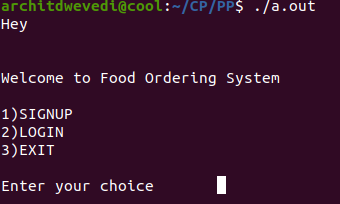Signup: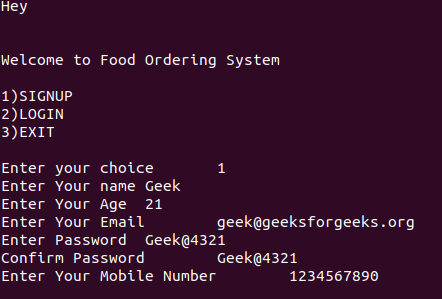Login: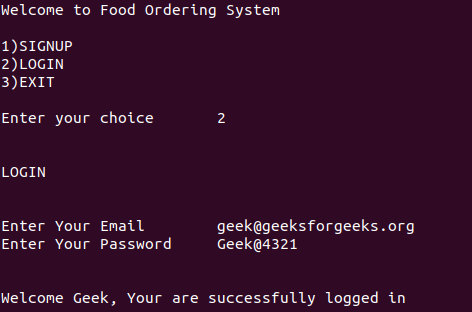Validate: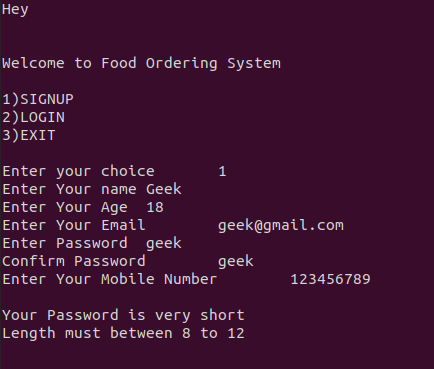Account Check: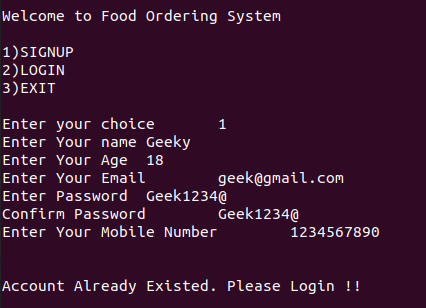Search By Hotels: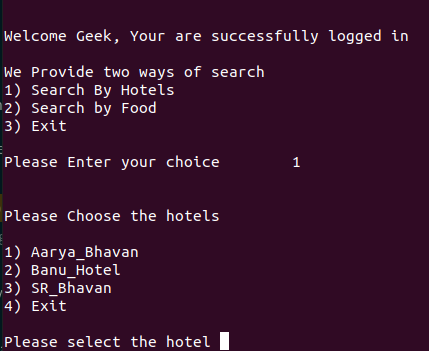Search By Food: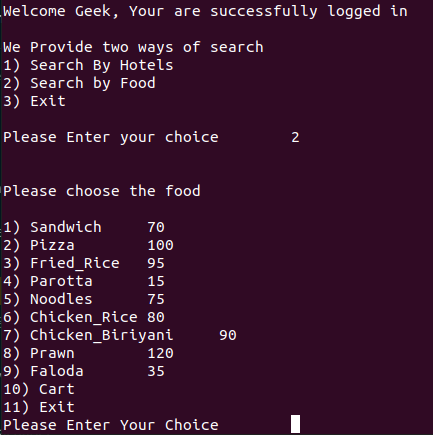Cart: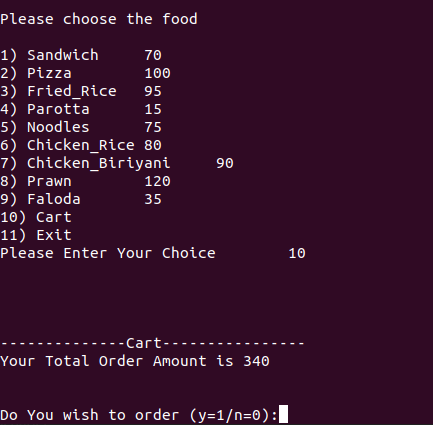Want to learn from the best curated videos and practice problems, check out the C Foundation Course for Basic to Advanced C.

My Personal Notes arrow_drop_up